# 十月末-solo博客

talk less,code more.

X

# 1 从物理模型开始

## 1.0 概念

• 衰减：H信道
• 干扰：n噪声

H^{-1}可以实现，但是n难以消除

### 衰落

• 大尺度衰落：主要由大型障碍物阴影衰落和信号的路径损耗造成，当移动台运动距离与小区尺寸相当时，会出现与频率无关的大尺度衰落
• 小尺度衰弱：多条信号路径的相长相消干扰造成，当空间尺度与载波波长相当时（相对距离较小），出现小尺度衰落，因此小尺度衰落与频率相关，比如多径效应多普勒效应

### 传播方式

• 直射：LOS-light of sight，视距传播，无遮挡的自由空间，如卫星通信
• 反射：reflection，物体尺度大于波长，如短波通信（大气电离层）
• 散射：scatter，电磁波照射到比载波波长小的物体上，发射出多路不同的较弱电磁波，如对流层散射信道
• 绕射：diffraction，照到物体不规则突出表面边缘反射，产生多个反射，如地波信道
• 透射：频率越高，穿透能力越差

### 噪声

• 外部噪声
• 近地噪声：天气
• 太阳系噪声，如日凌现象（所以不要把天线直射太阳）
• 宇宙噪声等
• 内部噪声
• 开关、低噪放、量化误差
• 热噪声（噪底），由实验器材、温度等决定，P_0=kTB，k-玻尔曼常数 $1.38e^{-23}J/K$

20MHz,20℃(293.15K)下接收机前端热噪声功率：

\begin{aligned}P_0&=1.38e^{-23}\times 293.15 \times 20e^{6}\\&\approx 8.09e^-14W\\&\approx-100.92dBm\end{aligned}

工业实用：
20℃，1Hz，-174dBm
20℃，1MHz，-114dBm
20℃，100MHz，-94dBm

• 白噪声：对各种噪声分布后按照中心极限定理的建模

## 1.1 自由空间

### 1.1.1 固定天线

E_s(f, t,(r, \theta, \psi))=\frac{\alpha_{s}(\theta, \psi, f) \cos 2 \pi f(t-r / c)}{r}

(r, \theta, \psi)是空间电场测量点ur是天线到点u的距离，\theta\psi是天线到u的垂直角度和水平角度，c是光速，\alpha_{s}(\theta, \psi, f)是天线以频率 f 在方向(\theta,\psi)的辐射方向图，包含了天线损耗的标量因子。电场相位随fr/c变化，对应光速辐射的时延。

u点有一副接收天线，假设噪声不存在，对于上述发射天线正弦信号的接收波形为：

E_r(f, t,(r, \theta, \psi))=\frac{\alpha(\theta, \psi, f) \cos 2 \pi f(t-r / c)}{r}

\alpha(\theta, \psi, f)是发射天线和接收天线在给定方向上的天线方向图之积。不妨把上述模型看作信道：

H(f):=\frac{\alpha(\theta, \psi, f) e^{-j 2 \pi f r / c}}{r}

### 1.1.2 运动天线

E_{r}\left(f, t,\left(r_{0}+v t, \theta, \psi\right)\right)=\frac{\alpha(\theta, \psi, f) \cos 2 \pi f\left[\left(1-\frac{v}{c}\right) t-\frac{r_{0}}{c}\right]}{r_{0}+v t}

### 自由空间传播损耗

LOS 的传播损耗：

P_R=\frac{{\lambda}^2}{(4\pi)^2d^2} P_TG_TG_R

\lambda-波长，d-距离，T-发射天线，R-接收天线，G-增益，P功率。记路径损耗为：P_{loss}=\frac{{\lambda}^2}{(4\pi)^2d^2}

\begin{aligned} Ploss&=10log(\frac{9e10^{16}}{16\pi^2\times 1e^6\times4e10^{18}})\\ &\approx 10log(2^{-6}e^{-8})\\ &\approx -80-3\times 6\\ &=-98dB \end{aligned}

## 1.2 单一垂直反射面

### 1.2.1 固定天线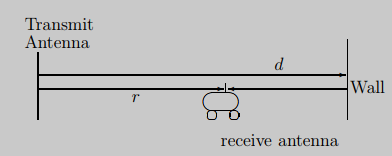E_{r}(f, t)=\frac{\alpha \cos 2 \pi f\left(t-\frac{r}{c}\right)}{r}-\frac{\alpha \cos 2 \pi f\left(t-\frac{2 d-r}{c}\right)}{2 d-r}

\Delta \theta=\left(\frac{2 \pi f(2 d-r)}{c}+\pi\right)-\left(\frac{2 \pi f r}{c}\right)=\frac{4 \pi f}{c}(d-r)+\pi

\Delta x_c=\frac{\lambda}{4}

\\\begin{aligned} \Delta\theta(r_1)-\Delta\theta(r_2)&=\pi \\\frac{4 \pi f}{c}(d-r1+r2)&=\pi\\d-r1+r2&=\frac{\lambda}{4}\end{aligned}

T_d=\frac{2d-r}{c}-\frac{r}{c}

\\\begin{aligned} \Delta\theta(f_1)-\Delta\theta(f_2)&=\pi \\ \frac{4\pi(d-r)}{c}(f_1-f_2)&=\pi\\ (f_1-f_2)^{-1}&=4\frac{(d-r)}{c}\\ (f_1-f_2)^{-1}&=2(\frac{2d-r}{c}-\frac{r}{c}) \end{aligned}

#时延扩展#是两条信号路径的传播时延之差。**如果如果信号频率该变量远小于 $1/T_d$，则特定时刻接收信号变化不明显。**

### 1.2.2 运动天线

E_{r}(f, t)=\frac{\alpha \cos 2 \pi f\left[\left(1-\frac{v}{c}\right) t-\frac{r_{0}}{c}\right]}{r_{0}+v t}-\frac{\alpha \cos 2 \pi f\left[\left(1+\frac{v}{c}\right) t+\frac{r_{0}-2 d}{c}\right]}{2 d-r_{0}-v t}

D_s\triangleq D_2-D_1=2f\frac{v}{c}

T_c=\frac{c}{4fv}

#相干时间#可以看作多普勒频移达到最大的时候来衡量，理解如下。

E_{r}(f, t) \approx \frac{2 \alpha \sin 2 \pi f\left[\frac{v}{c} t+\frac{\left(r_{0}-d\right)}{c}\right] \sin 2 \pi f\left[t-\frac{d}{c}\right]}{r_{0}+v t}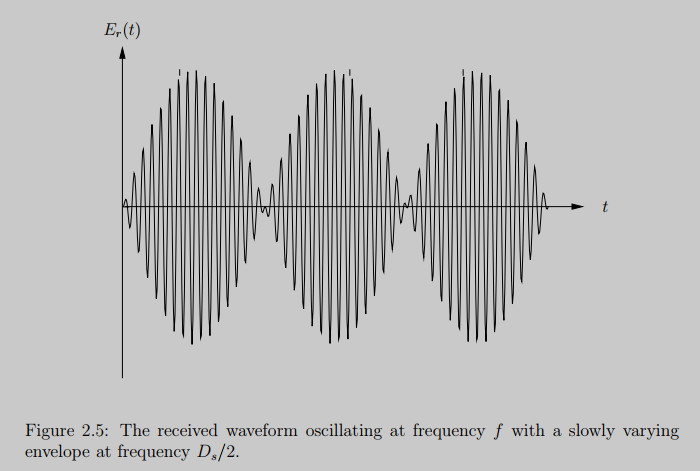### 反射会存在方向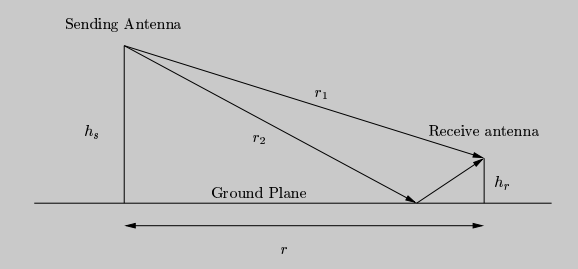## 1.3 阴影衰落

### 非自由空间的传播损耗

P_{R}= \begin{cases}\frac{\lambda^{2} P_{T} G_{T} G_{R}}{(4 \pi)^{2}} \times \frac{1}{d^{\alpha_{N}}}, & d<d_{b} \\ \left(h_{T} h_{R}\right)^{2} P_{T} G_{T} G_{R} \times \frac{1}{d^{\alpha_{F}}}, & d \geq d_{b}\end{cases}

d_b是传播断点，d_{b}=4 \pi h_{T} h_{R} \frac{1}{\lambda}d是传播距离。传播断点内衰减因子\alpha_N一般取 2；传播断点外的衰减因子\alpha_F取 3~5。

# 2 多径衰弱信道的输入输出模型

## 2.1 线性时变系统

y(t)=\sum_i{a_i(t)x(t-\tau_i(t))} \tag{1-1}

y(t)=\int_{-\infty}^{\infty} h(\tau, t) x(t-\tau) d \tau \tag{1-2}

h(\tau, t)=\sum_{i} a_{i}(t) \delta\left(\tau-\tau_{i}(t)\right) \tag{1-3}

h(\tau)=\sum_{i} a_{i} \delta\left(\tau-\tau_{i}\right) \tag{1-4}

## 2.2 基带等效模型

\begin{aligned} S_{b}(f)&=\left\{\begin{array}{cc} \sqrt{2} S\left(f+f_{c}\right) & f+f_{c}&gt;0 \\ 0 & f+f_{c} \leq 0 \end{array}\right. \\ \sqrt{2}S(f)&=S_b(f-fc)+S_b^*(-f-f_c) \end{aligned}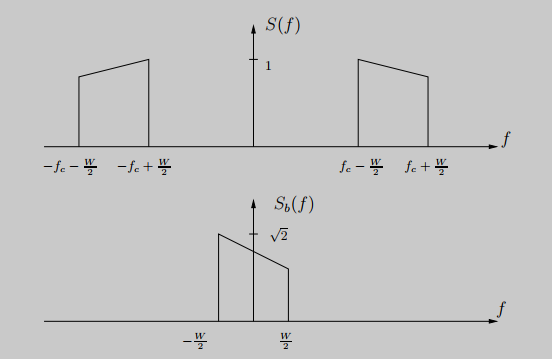y_{b}(t)=\sum_{i} a_{i}^{b}(t) x_{b}\left(t-\tau_{i}(t)\right) \tag{1-5}

h_{b}(\tau, t)=\sum_{i} a_{i}^{b}(t) \delta\left(\tau-\tau_{i}(t)\right) \tag{1-6}

T_c=\frac{1}{4D}

## 2.3 离散时间基带模型

x_b(t)=\sum_nx[n]sinc(Wt-n) \tag{1-7}

y_{b}(t)=\sum_{n} x[n] \sum_{i} a_{i}^{b}(t) \operatorname{sinc}\left(W t-W \tau_{i}(t)-n\right) \tag{1-8}

\begin{aligned} y[m]&=\sum_{n} x[n] \sum_{i} a_{i}^{b}(m / W) \operatorname{sinc}\left[m-n-\tau_{i}(m / W) W\right]\\ &\underset{l=m-n}{=}\sum_{\ell} x[m-\ell] \sum_{i} a_{i}^{b}(m / W) \operatorname{sinc}\left[\ell-\tau_{i}(m / W) W\right]\\ &=\sum_l{h_l[m]x[m-l]} \end{aligned} \tag{1-9}

h_{\ell}=\sum_{i} a_{i}^{b} \operatorname{sinc}\left[\ell-\tau_{i} W\right] \tag{1-10}

LTI 情况下第l个抽头可以理解为"(1-6)"5卷积 sinc 的第\frac{l}{W}个样本，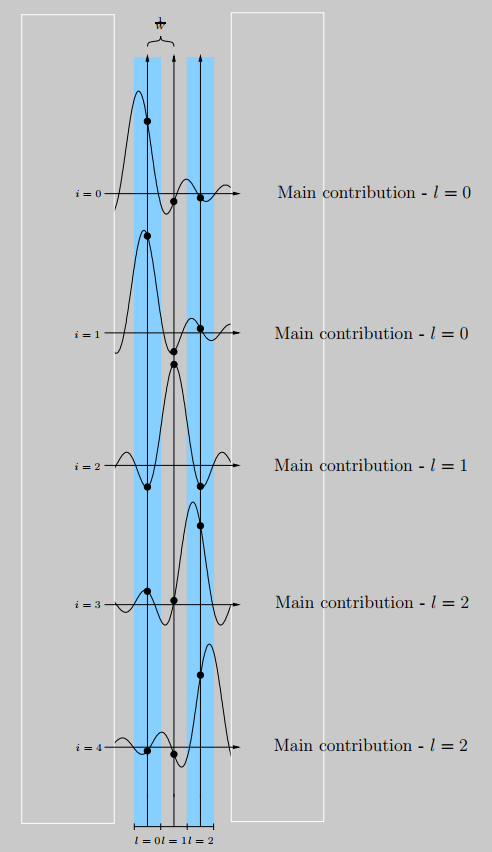## 2.4 数字通信系统系统框图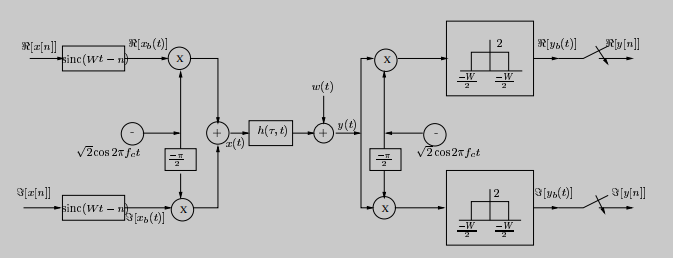IQ 两路复码元-> 调制 sinc 脉冲-> 上变频-> 过信道-> 下变频-> 低通滤波->\frac{m}{W}时刻采样。

AWGN 的假设实质上意味着主要的噪声源位于接收机，或者说噪声源是进入接收机与接收信号路径相互独立的辐射 。

# 3 信道分类与统计模型

## 3.1 重要概念

### #多普勒扩展#与#相干时间#

D_{s}:=\max _{i, j} f_{c}\left|\tau_{i}^{\prime}(t)-\tau_{j}^{\prime}(t)\right| \tag{1-11}

T_c\approx\frac{1}{4D_s} \tag{1-12}

### #时延扩展#与#相干带宽#

T_{d}:=\max _{i, j}\left|\tau_{i}(t)-\tau_{j}(t)\right| \tag{1-13}

#时延扩展#控制了#相干带宽#，#相干带宽#表明信道随频率变化的快慢。将"一、1.2.1"10的概念推广到任意数量的路径，#相干带宽#Wc为：

W_c=\frac{1}{2T_d} \tag{1-14}

• #相干时间#表明了信道随时间变化的快慢
• #相干带宽#表明了信道随频率变化的快慢

## 3.2 信道分类

### 3.2.1 快衰落与慢衰落

• #相干时间#T_c小于应用的时延需求，称信道快衰落
• #相干时间#T_c大于应用的时延需求，称信道为慢衰落

1. 快衰弱和慢衰落是相对应用时延需求而言的，不是孤立地说信道是快衰落或者慢衰落。
2. 相干时间反应的是信道抽头相位重大变化的时间，如果应用的时延需求比信道抽头变化的时间小，对于短时间内通信而言信道变化相对得慢；如果信道变化比应用的时延需求小，对于短时间内通信而言信道变化相对得快。

### 3.2.2 频率选择性与平坦衰落

• #相干带宽#W_c远小于系统输入带宽，称信道为频率选择性
• #相干带宽#W_c大于系统输入带宽，称信道为平坦衰落

1. 频率选择性和平坦衰落是相对系统输入带宽而言的，不能孤立地说信道是频率选择性或平坦衰落
2. 频率在#相干带宽#内的信号过信道后变化慢，在#相干带宽#外的信号过信道后变化快。

### 3.2.3 欠扩展

T_d\ll T_c为欠扩展信道，这是在蜂窝通信中是常见的。

## 3.3 信道抽头统计模型

### 3.3.2 莱斯模型

h_{\ell}[m]=\sqrt{\frac{\kappa}{\kappa+1}} \sigma_{\ell} e^{j \theta}+\sqrt{\frac{1}{\kappa+1}} \mathcal{C} \mathcal{N}\left(0, \sigma_{\ell}^{2}\right)

\kappa是镜像路径能量与散射路径能量之比，\kappa越大说明信道的确定性越强（镜像路径能量越大）。

### 3.3.3 抽头自相关函数

R_{\ell}[n]:=\mathbb{E}\left\{h_{\ell}^{*}[m] h_{\ell}[m+n]\right\}

T_d=\frac{1}{W}\sum_lR_l

### 3.3.4 克拉克模型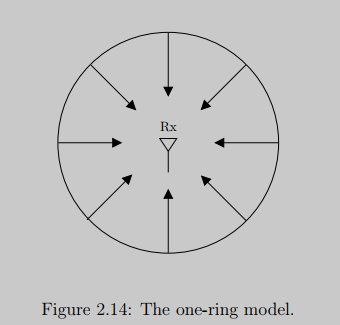y[m]=h_{0}[m] x[m]+w[m] \\ h_0[m]\sim \mathcal{CN}

S(f)=\left\{\begin{array}{cc} \frac{2 a^{2}}{D_{s} \sqrt{1-\left(2 f / D_{s}\right)^{2}}} & -D_{s} / 2 \leq f \leq+D_{s} / 2 \\ 0 & \text { else } \end{array}\right.• 克拉克模型得到的信道是瑞利模型 + 平坦衰落，抽头数为 1 且服从复高斯分布。
• S(f)在最大多普勒频移以外为 0，又称多普勒谱。
y(t)=\sum_i{a_i(t)x(t-\tau_i(t))} \tag{1-1}
y(t)=\int_{-\infty}^{\infty} h(\tau, t) x(t-\tau) d \tau \tag{1-2}
h(\tau, t)=\sum_{i} a_{i}(t) \delta\left(\tau-\tau_{i}(t)\right) \tag{1-3}
y_{b}(t)=\sum_{i} a_{i}^{b}(t) x_{b}\left(t-\tau_{i}(t)\right) \tag{1-5}
h_{b}(\tau, t)=\sum_{i} a_{i}^{b}(t) \delta\left(\tau-\tau_{i}(t)\right) \tag{1-6}
x_b(t)=\sum_nx[n]sinc(Wt-n) \tag{1-7}
\begin{aligned} y[m]&amp;=\sum_{n} x[n] \sum_{i} a_{i}^{b}(m / W) \operatorname{sinc}\left[m-n-\tau_{i}(m / W) W\right]\\ &amp;\underset{l=m-n}{=}\sum_{\ell} x[m-\ell] \sum_{i} a_{i}^{b}(m / W) \operatorname{sinc}\left[\ell-\tau_{i}(m / W) W\right]\\ &amp;=\sum_l{h_l[m]x[m-l]} \end{aligned} \tag{1-9}

1. 像之前一样，在固定距离的时候，考虑相位变化，从波峰到波谷经历的时间为称为#相干时间#（coherenc time）：

2. 从"（1-5）"1和"（1-6）"1可以看出：基带出说为各路径基带输入的时延副本之和，第i项幅度为第i条路径的响应幅度。当a_{i}^{b}(t)的路径时延\tau_i(t)变化\frac{1}{4f_c}时，相位改变\frac{\pi }{2}——如果路径长度以速度v变化那么相位变化所需时间为\frac{c}{4fv}，这正是"之前"1计算的#相干时间#。相干时间T_c与多普勒频移D（不是多普勒扩展）关系为: